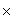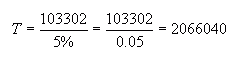From: Mike How would I calculate a reverse percentage? Let me give you an example.  I have two values that calculate to a success rate of 93.32.  Total=1546051 Failures=103302.  (1546051-103302)/1546051=93.32% success .   Now how much would I need to increase the Total value to get the success up to 95%? Thank You. Hi Mike. A 95% success rate is the same as a 5% failure rate. You know the number of failures (that's 103302), but you need to know the total. So let T = the total you need. 5%T = 103302 So let's re-arrange this to solve for T:This is the new total you need that will allow 103302 to be 5%. Subtract your original total from this new total to get the amount by which you need to increase your total (without adding any more failures!) to get up to a 95% sucess rate. Hope this helps! Sue